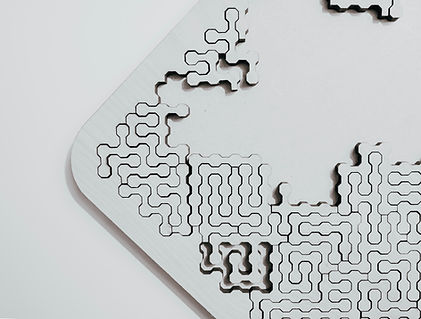top of pageCalcudoku

## Instructions

Calcudoku puzzles, also known under the trademarked name KenKen, are a fun blend of logic and arithmetic. A Calcudoku puzzle uses the numbers 1 through 4 for a 4 by 4 puzzle, 1 through 5 for a 5 by 5 puzzle, and so on. Like Sudoku, each row and column must contain each of the numbers for the puzzle exactly once creating what mathematicians call a Latin square. Unlike a Sudoku puzzle, none of the numbers are provided, you will need to use logic to place the numbers in each cell according to the clues given in each “cage”.

Addition (+) or multiplication (×) clues describe the sum or product of the caged numbers. Subtraction (−) or division (÷) clues describe the difference or quotient of the larger number by the smaller number in the cage (which always has two cells). Numbers can be repeated within a cage, as long as they don’t share a row or column.

The puzzle provided is a 7 x 7 grid, it should be filled in using numbers 1 through 7. Enjoy this week's puzzle fun.